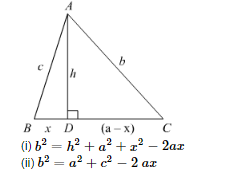# In the given figure, ∠B < 90° and segment AD ⊥ BC, show that

Question:

In the given figure, $\angle \mathrm{B}<90^{\circ}$ and segment $\mathrm{AD} \perp \mathrm{BC}$, show thatSolution:

(i) Since AD perpendicular to BC we obtained two right angled triangles, triangle ADB and triangle ADC.

We will use Pythagoras theorem in the right angled triangle ADC

$A C^{2}=A D^{2}+D C^{2}$.....(1)

Let us substitute AD = h, AC = b and DC = (a − x) in equation (1) we get,

$b^{2}=h^{2}+(a-x)^{2}$

$b^{2}=h^{2}+a^{2}-2 a x+x^{2}$

$b^{2}=h^{2}+a^{2}+x^{2}-2 a x$.....(2)

(ii) Let us use Pythagoras theorem in the right angled triangle ADB as shown below,

$A B^{2}=A D^{2}+B D^{2}$

Let us substitute AB = c, AD = h and BD = x in equation (3) we get,

$c^{2}=h^{2}+x^{2}$

Let us rewrite the equation (2) as below,

$b^{2}=h^{2}+x^{2}+a^{2}-2 a x$....(4)

Now we will substitute $h^{2}+x^{2}=c^{2}$ in equation (4) we get,

$b^{2}=c^{2}+a^{2}-2 a x$

Therefore, $b^{2}=c^{2}+a^{2}-2 a x$.